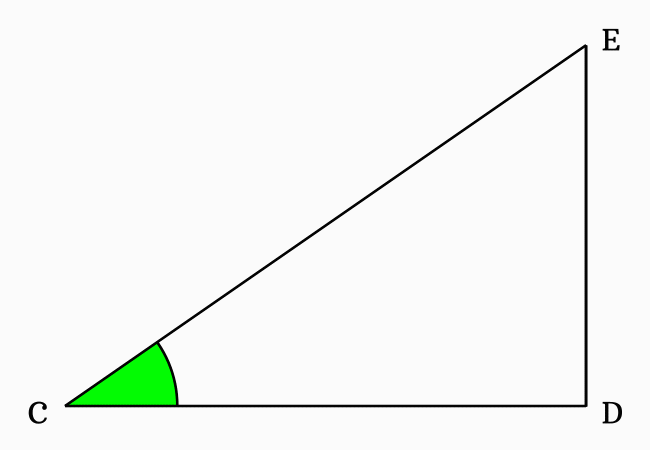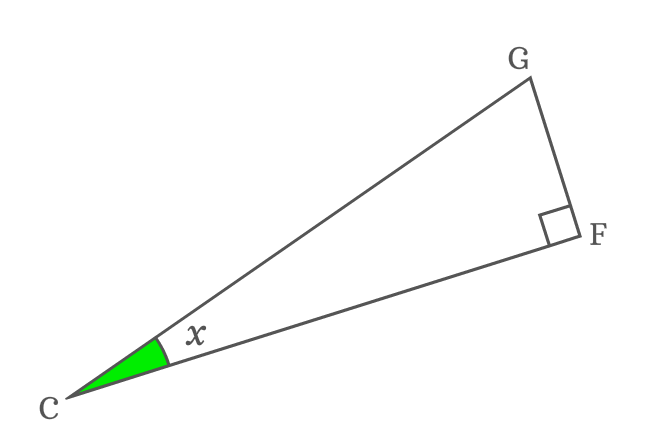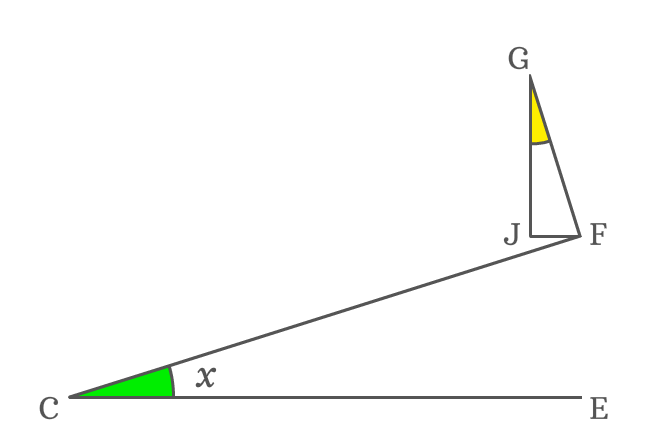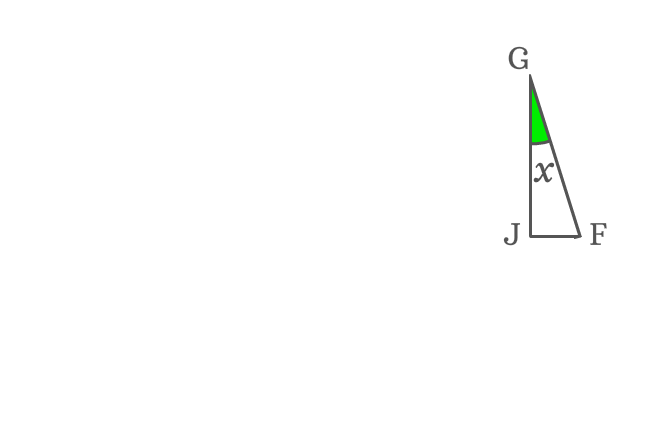# Proof of sin double angle formula

The sin double angle identity is a double angle trigonometric identity and it is used as a formula in trigonometry to expand sin double angle functions such as $\sin{2x}$, $\sin{2\theta}$, $\sin{2A}$, $\sin{2\alpha}$ and etc. The expansion of sin double angle formula is actually derived in geometric system. Now, it is your time to learn how to derive sin double angle identity geometrically.

## Construction

The sin of double angle identity is derived geometrically by constructing a right triangle with double angle. In this case, the right triangle (represented by $\Delta DCE$) with double angle is constructed geometrically by the following four steps.1. Draw a line from point $\small C$ to side $\small \overline{DE}$ to bisect the $\small \angle DCE$ and it intersects the side $\small \overline{DE}$ at point $\small F$. Take, each angle is represented by $x$.
2. Draw a line to side $\small \overline{CD}$ from point $\small F$ but it should be perpendicular to side $\small \overline{CF}$ and take that it intersects the side $\small \overline{CD}$ at point $\small G$.
3. Draw a perpendicular line to side $\small \overline{CE}$ from point $\small G$. It intersects the side $\small \overline{CF}$ at point $\small H$ and perpendicularly intersects side $\small \overline{CE}$ at point $\small I$.
4. Draw a perpendicular line to side $\small \overline{GI}$ from point $\small F$ and it intersects the side $\small \overline{GI}$ at point $\small J$. Similarly, draw a perpendicular line to side $\small \overline{DE}$ from point $\small H$ and it intersects the side $\small \overline{DE}$ at point $\small K$.

### Proof

A right triangle with double angle is constructed geometrically and it is used to derive the expansion of sin double angle identity in trigonometric form.

#### Express sine of double angle

The side $\overline{CF}$ bisects the $\angle DCE$ as two equal angles $\angle ECF$ and $\angle FCD$. If $\angle ECF$ is denoted by $x$, then $\angle FCD$ is also equal to $x$.$\angle DCE = \angle ECF + \angle FCD$

$\implies \angle DCE = x + x$

$\implies \angle DCE = 2x$

The $\angle DCE$ and $\angle ICG$ are congruent. Therefore, $\angle ICG = \angle DCE = 2x$.

Now, write sin of double angle ($\sin{2x}$) in its trigonometric ratio form as per $\Delta ICG$.

$\sin{2x} \,=\, \dfrac{GI}{CG}$

The side $\overline{JF}$ splits the side $\overline{GI}$ as two sides $\overline{GJ}$ and $\overline{JI}$. So, the length of side $\overline{GI}$ can be written as the sum of them mathematically.$GI = GJ + JI$

Now, replace the length of side $\overline{GI}$ by its equivalent value in $\sin{(2x)}$ expansion.

$\implies$ $\sin{2x} \,=\, \dfrac{GJ+JI}{CG}$

$\implies$ $\sin{2x} \,=\, \dfrac{GJ}{CG}+\dfrac{JI}{CG}$

The sides $\overline{GI}$ and $\overline{DE}$ are parallel lines. So, the length of the side $\overline{JI}$ is exactly equal to the length of the side $\overline{EF}$. Therefore, $JI = EF$.

$\implies$ $\sin{2x} \,=\, \dfrac{GJ}{CG}+\dfrac{EF}{CG}$

#### Express trigonometric ratios in functions

The $\sin{(2x)}$ is expanded in terms of the ratios between the sides. Now, let’s try to express each ratio in the form a trigonometric function.

The side $\overline{EF}$ is opposite side of the $\Delta ECF$ and its angle is $x$. Try to write the length of side $\overline{EF}$ in terms of a trigonometric function.$\sin{x} = \dfrac{EF}{CF}$

$\implies EF = {CF}\sin{x}$

Now, substitute the length of perpendicular $\overline{EF}$ by its equivalent value in the expansion of $\sin{2x}$.

$\implies$ $\sin{2x} \,=\, \dfrac{GJ}{CG}+\dfrac{{CF}\sin{x}}{CG}$

$\implies$ $\sin{2x} \,=\, \dfrac{GJ}{CG}+\dfrac{CF}{CG} \times \sin{x}$

The sides $\overline{CF}$ and $\overline{CG}$ are sides of the right triangle $GCF$ and the angle of this triangle is also $x$. According to trigonometry, the ratio of them can be represented by $\cos{x}$.$\cos{x} = \dfrac{CF}{CG}$

Now, replace the ratio of lengths of sides $\overline{CF}$ to $\overline{CG}$ by its equivalent trigonometric function in the expansion of sin of double angle function.

$\implies$ $\sin{2x} \,=\, \dfrac{GJ}{CG}+\cos{x} \times \sin{x}$

$\implies$ $\sin{2x} \,=\, \dfrac{GJ}{CG}+\cos{x}\sin{x}$

$\implies$ $\sin{2x} \,=\, \dfrac{GJ}{CG}+\sin{x}\cos{x}$

#### Geometric Analysis for unknown angle

The side $\overline{GJ}$ is one of the sides of the $\Delta JGF$ but its angle is unknown. So, it is not possible to express the length of the side $\overline{GJ}$ in terms of a trigonometric function. Hence, let’s find the angle of this triangle geometrically.

The sides $\overline{CE}$ and $\overline{JF}$ are parallel lines and $\overline{CF}$ is their transversal. $\angle ECF$ and $\angle JFC$ are interior alternate angles and they are equal geometrically.$\angle JFC = \angle ECF = x$

The side $\overline{FG}$ is a perpendicular line to side $\overline{CF}$. So, the $\angle CFG = 90^°$. but the side $\overline{JF}$ splits the $\angle CFG$ as $\angle JFC$ and $\angle JFG$.

$\angle JFC + \angle JFG = 90^°$

It is proved about that $\angle JFC = x$.

$\implies x + \angle JFG = 90^°$

$\implies \angle JFG = 90^°-x$

$\Delta JGF$ is a right triangle in which $\angle GJF = 90^°$ and $\angle JFG = 90^°-x$. The $\angle JGF$ can be calculated by the sum of angles rule of a triangle.

$\angle JGF + \angle GJF + \angle JFG$ $=$ $180^°$

$\implies \angle JGF + 90^° + 90^°-x$ $=$ $180^°$

$\implies \angle JGF + 180^°-x$ $=$ $180^°$

$\implies \angle JGF$ $=$ $180^°-180^°+x$

$\,\,\, \therefore \,\,\,\,\,\, \angle JGF = x$

It is proved that the $\angle JGF$ is also equal to $x$ because it is a similar triangle.

#### Write trigonometric ratio in functions

$\sin{2x} \,=\, \dfrac{GJ}{CG}+\sin{x}\cos{x}$

Now, let’s try to write the ratio between sides $\overline{GJ}$ and $\overline{CG}$ in terms of trigonometric functions to get the expansion of sin double angle identity.$\overline{GJ}$ is adjacent side of $\Delta JGF$ and its angle is derived as $x$.

$\cos{x} = \dfrac{GJ}{GF}$

$\implies GJ = {GF}\cos{x}$

Substitute the length of $\overline{GJ}$ by its equivalent value in the expansion of $\sin{(2x)}$ function.

$\sin{2x}$ $\,=\,$ $\dfrac{{GF}\cos{x}}{CG}+\sin{x}\cos{x}$

$\implies \sin{2x}$ $\,=\,$ $\dfrac{GF}{CG} \times \cos{x}+\sin{x}\cos{x}$

The sides $\overline{GF}$ and $\overline{CG}$ are sides of $\Delta GFC$. So, consider right triangle $GFC$ once more time.$\sin{x} = \dfrac{GF}{CG}$

Therefore, replace the ratio of lengths of sides $\overline{GF}$ to $\overline{CG}$ by trigonometric function $\sin{x}$ in the $\sin{2x}$ expansion.

$\implies \sin{2x}$ $\,=\,$ $\sin{x} \times \cos{x}+\sin{x}\cos{x}$

$\implies \sin{2x}$ $\,=\,$ $\sin{x}\cos{x}+\sin{x}\cos{x}$

$\,\,\, \therefore \,\,\,\,\,\, \sin{2x}$ $\,=\,$ $2\sin{x}\cos{x}$

Therefore, it is proved geometrically that the sin of double angle function can be expanded as two times the product of sin of angle and cos of angle.

Email subscription
Math Doubts is a best place to learn mathematics and from basics to advanced scientific level for students, teachers and researchers. Know more